# XLOOKUPArticle byJeevan A Y## XLOOKUP Function Definition

XLOOKUP looks for a specific value in a range of cells or a table array and returns the value from another column with an exact, partial, or approximate match. Furthermore, it allows users to search for the desired value from the horizontal and vertical data sets.

For instance, we can use the XLOOKUP function to find an employee’s department based on their ID using the data in the excel sheet.

Firstly, we will create an employee ID reference cell in E1 containing ID144.

Next, we will try to retrieve the department for the employee ID, ID144, by entering the below formula in cell F1.

=XLOOKUP(E1,B2:B11,A2:A11,,0)

XLOOKUP function fetches the department name from A2:A11 based on the employee ID reference in cell E1 and lookup array reference of B2:B11. XLOOKUP returns the result as “Finance,” which is the department for the employee ID, ID144.

We can use the  XLOOKUP in excel to get the desired result for the lookup value.

###### Key Takeaways
• XLOOKUP searches a range of cells or a table array for a given value and returns an exact, partial, or approximate match from another column horizontally and vertically.
• In Excel, XLOOKUP is a more advanced form of VLOOKUP and HLOOKUP. It outperforms VLOOKUP in terms of flexibility and variety.
• XLOOKUP performs two-way match-up by using nested XLOOKUP functions. For example, it can fetch values from left to right and right to left.
• It is a new function available only for the Microsoft Office 365 version. For a single lookup value, XLOOKUP can return multiple values.

### XLOOKUP Function Syntax

Below is the the XLOOKUP formula in excel:

The syntax for XLOOKUP looks similar to VLOOKUP with additional parameters. XLOOKUP has six parameters where three are mandatory, and the remaining three are optional.

• Lookup_value: The value for which we are trying to retrieve the result from the return array (3rd argument). It is a mandatory argument.
• Lookup_array: This will be either range of cells or a table array where we search for the lookup value. It is also a mandatory argument.
• Return_array: This is the array from which we need to get the desired result for the lookup value provided in the first argument. It is also a mandatory argument.
• [If_not_found]: If the lookup value is not in the lookup array, it results in a #N/A error. However, we can provide and get an alternative result here instead of the default #N/A error. This is an optional argument.
• [Match_Type]: In this argument, we need to specify the kind of match we need to do:
• 0 – It will search for the exact match of the lookup value in the lookup array. If nothing is specified, 0 will be the default input.
• -1 – This will look for an exact match. But if not found, it will look for the next smaller item than the lookup value.
• 1 – This looks for an exact match. But if not found, it will look for the next larger item than the lookup value.
• 2 – This will perform a partial match using wildcard characters, like an asterisk (*) and tilt (~).
• [Search_Mode]: In this argument, we specify how XLOOKUP searches for the lookup value:
• 1 – This is the default searching option. It will allow the XLOOKUP to start searching from top to bottom in the lookup array.
• -1 – This will do the searching from bottom to top. It is helpful to find the last matching result for the lookup value.
• 2 – This will help us do binary searching for the data sorted in ascending order. If not sorted, we will get either the wrong result or an error.
• -2 – This will help us do binary searching for the data sorted in descending order. If not sorted, we will get either the wrong result or an error.

### How To Use XLOOKUP?

Let us have a look at a basic XLOOKUP example:

Below is the data set of employees and the wages they earned across months.

We will try to get the wages for the employee ID, ID123 for March, using the XLOOKUP function from the above data set.

Here are the steps to use XLOOKUP() Excel Function –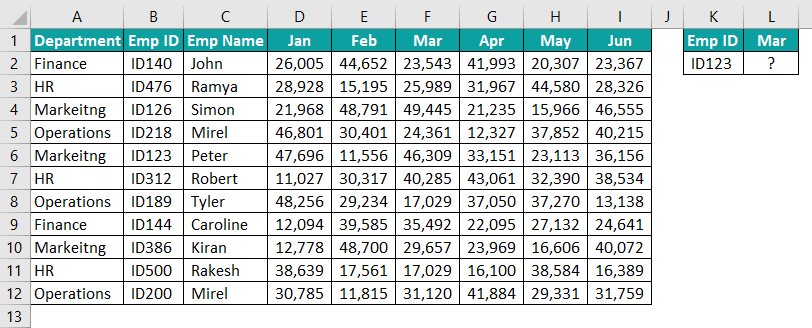1. Create an employee ID reference cell in K2 containing ID123. Enter the XLOOKUP function in cell L2.2. The first argument is lookup value, i.e., ID123 in the reference cell K2. Select this cell.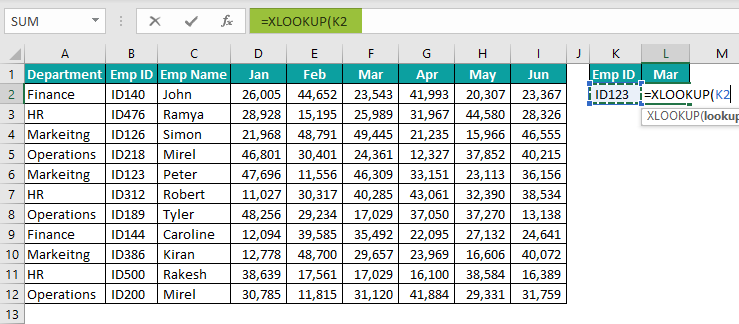3. Next, we will select the lookup array, i.e., Emp ID array B2:B12, in the table.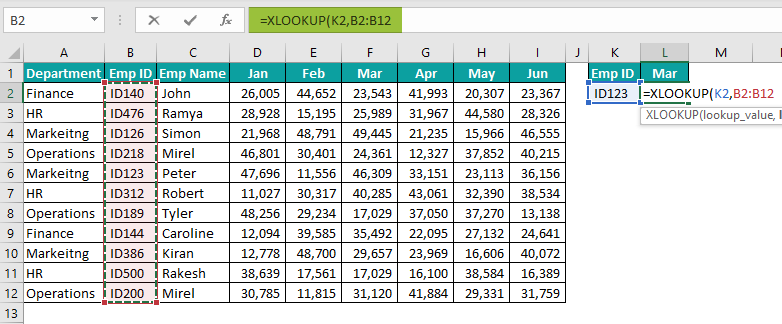4. Next, we look for the return array, i.e., wages for March, so select the column array F2:F12.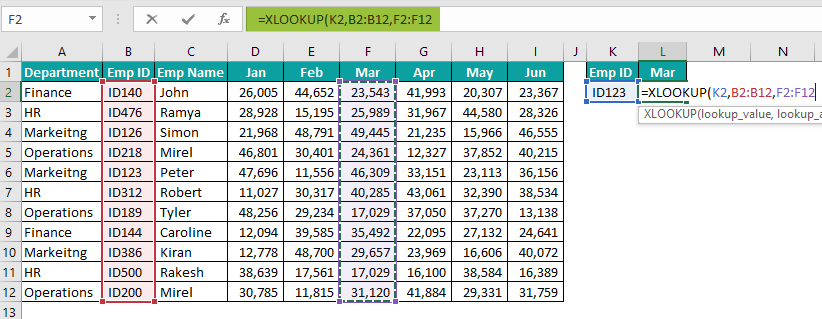As of now, ignore the last three optional arguments, close the bracket, and hit the “Enter” key to get the result.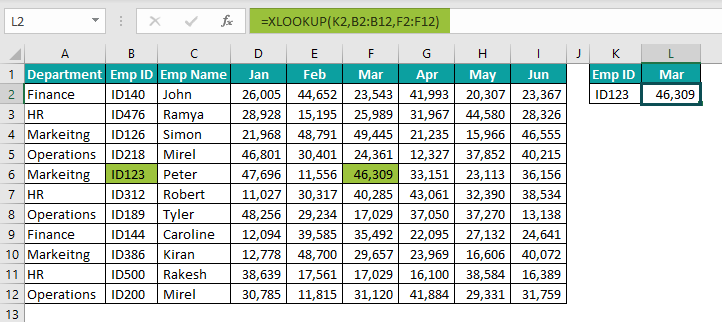The result is 46,309, i.e., the wages for the Emp ID, ID123 for March. In this way, we can use the XLOOKUP function to retrieve the values from the data set based on the lookup value given.

### Examples

Let us look at some advanced XLOOKUP examples to understand how it works:

#### Example #1: Two-Way Lookup

In the previous example, we selected the result array for March. Still, if we want to look for another month, e.g., February, we must manually change the result array selection.

We can make this dynamic by using a two-way lookup.

Step 1: Enter the XLOOKUP function in cell L2.

Step 2: Here, we are performing XLOOKUP two-way lookup, so first, we need to get the month’s position in the data set. Hence, select the lookup value as the month, i.e., cell L1.

Step 3: Next, we will select the lookup array D1:I1.

Step 4: The above formula will return the row number for the given month in cell L2, i.e., Feb.

Next, we will enter another XLOOKUP function within the existing XLOOKUP formula in the return array argument.

Step 5: For this XLOOKUP, we will select the lookup value as Emp ID in cell K2.

Step 6: In the next argument lookup array, we will choose the array B2:B12.

Step 7: Next, we will choose the array D2:I12 as the return array.

This will conclude our two-way lookup formula, close the brackets, and hit the ‘Enter’ key to get the result.

The wages for the emp ID, ID123, and February are 11,556.

The dynamic nature of this two-way lookup is we can change the Emp ID and month name, and the formula will return the result for the given Emp ID and month.

For instance, we will change Emp ID to 189 and month name to May.

In this way, we can use the XLOOKUP function to perform the two-way lookup.

#### Example #2: Retrieve the Entire Row Record for the Lookup Value

In the previous example, we learned how to fetch value for a single month. However, we can also use the XLOOKUP function to get the entire row record for a specific employee.

Let us use the same data for this example. Again, we will try to retrieve all the month’s wages for a specific employee this time.

Step 1: Enter the XLOOUP function in cell B15.

Step 2: Choose the lookup value as cell A15.

Step 3: Choose lookup array B2:B12, where we will search for cell A15 Emp ID.

Step 4: Next, we will choose the month array, i.e., D2:I12, for the result array.

Step 5: Ignore optional arguments. Close the bracket and hit the ‘Enter’ key to get the result.

The above formula uses the return array as D2:D12 instead of one single column. And XLOOKUP returns all the rows for the lookup value in cell A15.

Another specialty of this operation is that we cannot delete the formula in cells other than B15. If we try, nothing will happen in cells D15:G15. However, if we delete the formula in cell B15, the formula will be deleted in all the cells.

In this way, XLOOKUP is more versatile than VLOOKUP, in which we need to enter the column numbers for each month separately.

#### Example #3: Find the Last Matching Value

Unlike VLOOKUP, which searches from top to bottom, we can use the XLOOKUP function to retrieve the value from bottom to top.

For instance, look at the below data set.

In the above data set, we have employee “Mirel” two times but with two different emp IDs. So the objective here is to find the emp ID for the last occurrence, “Mirel,” i.e., cell B12 value.

Apply the same steps that we have applied until the return type.

We will ignore the next two arguments, i.e., If_not_found and match_mode, by entering three commas.

In the search mode argument, we will choose -1, which will search from bottom to top.

Close the bracket and hit the ‘Enter’ key to get the result.

The emp ID for “Mirel” is ID200.

This is because of the last argument we have given as -1, which searches value from bottom to top rather than the usual one of the top to bottom.

#### Example #4: Horizontal Lookup

For horizontal lookups, we have used HLOOKUP all this while. However, we can use XLOOKUP going forward.

We have horizontally transformed the above data.

We will try to retrieve the wages for the Emp ID, ID140, and Apr.

Step 1: Enter the XLOOKUP function in cell B12.

Step 2: Enter lookup value as cell B11.

Step 3: Next, we will choose the lookup array from B2:L2.

Step 4: Next, we will select the return array from B7:L7 because we are looking for Apr wages.

Step 5: Close the bracket and hit the ‘Enter’ key to get the result.

The result in cell B12 is 41,993, which is for the emp ID, ID140.

In this way, XLOOKUP is flexible and versatile to assist horizontal and vertical lookups.

### Important Things To Note

• XLOOKUP is available in Microsoft Office 365 version only.
• It can retrieve the value from both horizontal and vertical datasets.
• Users can get alternative results in case of errors by using the If_not_found argument.
• By default, XLOOKUP looks for the exact match and does not require mentioning the match_type argument as 0.
• XLOOKUP can retrieve the values from left to the right, right to left, top to bottom, and bottom to top. Hence, the order of the data is not a matter of concern.

What is XLOOKUP in excel with an example?

XLOOKUP is a new function in Microsoft Office 365, which searches for a given value within a range of cells or a table array and returns the value from another column: an exact, partial, or approximate match. It allows users to search the horizontal and vertical data sets for the desired value. Furthermore, it assists in the top to bottom, bottom to top, two-way lookup, and returning an entire row or column of data.

Below is a spreadsheet containing students and their scores in an examination.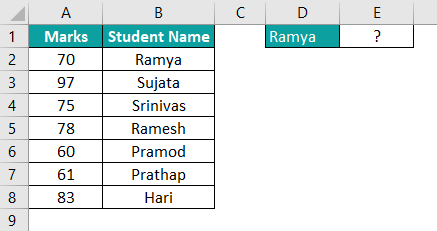We will use the XLOOKUP function to fetch the score of “Ramya” in cell E1.

Enter the XLOOKUP function in cell E1 and copy-paste the below formula.

=XLOOKUP(D1,B2:B8,A2:A8)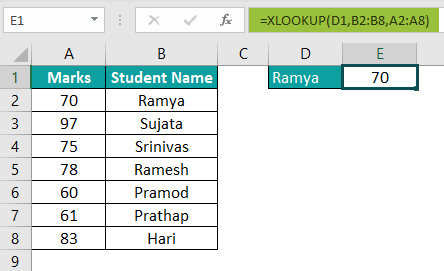XLOOKUP returned the result as 70, the student “Ramya” score.

Here, XLOOKUP looks for the lookup value “Ramya” (D1 cell) in lookup array B2:B8 and returns the corresponding row value in return array A2:A8.

Is XLOOKUP better and faster than the VLOOKUP?

XLOOKUP is not faster than VLOOKUP on large data sets. However, it is the advanced version of VLOOKUP and HLOOKUP in excel. In addition, XLOOKUP is much better than VLOOKUP in flexibility and versatility, as it retrieves values from left to right and right to left.

Can XLOOKUP return multiple values?

Yes, XLOOKUP can return multiple values for a single lookup value. For example, it can return the entire row or column value for the desired lookup value of the return array.

How do I enable XLOOKUP in Excel?

XLOOKUP is available for Office 365 users. So, if you are using 2019 and earlier versions, you will not be able to use this.

If you already use Office 365 and are still not able to use it, you will have to enable it to start using it. Follow the below steps:

File >>> Account >>> Office Insider.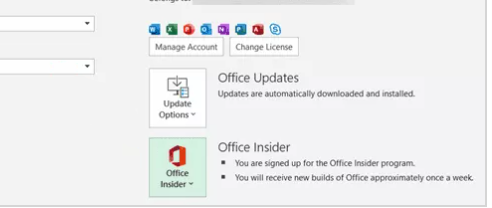Join Office Insider Program, and this will give you access to the XLOOKUP function.